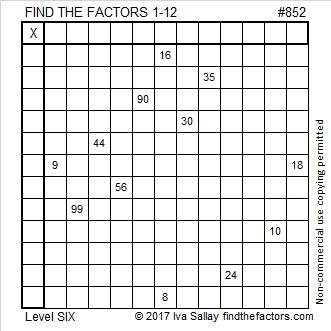# 852 and Level 6Print the puzzles or type the solution on this excel file: 12 factors 843-852

I knew that 852 was divisible by 3 as soon as I typed it in a straight line on the number pad. Any 3 digit number that lies on a straight line on a number pad or a phone dial pad is divisible by 3. And in case you’ve ever wondered why the numbers on a number pad or calculator and the numbers on a phone dial pad are reversed, ABC News has the answer.852 is 705 in BASE 11, and it is 507 in BASE 13.

852 is palindrome 1E1 in BASE 23 (E is 14 base 10) because 1(23²) +14(23¹) + 1(23º) = 852.

852 is the sum of consecutive prime numbers 421 and 431.

852 is also the 24th pentagonal number because (3⋅24² – 24)/2 = 852

• 852 is a composite number.
• Prime factorization: 852 = 2 × 2 × 3 × 71, which can be written 852 = 2² × 3 × 71
• The exponents in the prime factorization are 2, 1, and 1. Adding one to each and multiplying we get (2 + 1)(1 + 1)(1 + 1) = 3 × 2 × 2 = 12. Therefore 852 has exactly 12 factors.
• Factors of 852: 1, 2, 3, 4, 6, 12, 71, 142, 213, 284, 426, 852
• Factor pairs: 852 = 1 × 852, 2 × 426, 3 × 284, 4 × 213, 6 × 142, or 12 × 71,
• Taking the factor pair with the largest square number factor, we get √852 = (√4)(√213) = 2√213 ≈ 29.189039